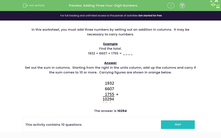### Comprehensive & curriculum aligned

In this worksheet, students add three 4-digit whole numbers by setting out their addition in columns. It may be necessary to carry numbers.Key stage:  KS 2

Curriculum topic:   Number: Addition and Subtraction

Curriculum subtopic:   Add/Subtract up to Four-Digit Numbers

Difficulty level:#### Worksheet Overview

In this worksheet, you must add three numbers by setting out an addition in columns.  It may be necessary to carry numbers.

Example

Find the total.

1932 + 6607 + 1755 = ____

Set out the sum in columns.  Starting from the right in the units column, add up the columns and carry if the sum comes to 10 or more.  Carrying figures are shown in orange below.